## Reynold Number for Drilling Hydraulics

Reynold number is the important figure because it demonstrates the flow regimes of drilling mud as laminar, transition or turbulent flow in annulus of the wellbore. In order to correctly calculate the Reynolds number, you need to use the effective viscosity, µea, which we already discuss about it from the previous topic.

The following equation is for Reynolds number in the annulus.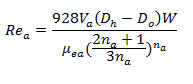Where:

Rea = Reynold Number in the annulus

Va = Annular velocity, ft/min

Dh = Diameter of wellbore, inch

Do = Outside Diameter of tubular, inch

W = mud weight, ppg

µea = effective viscosity in the annulus, centi-poise

na = power law constant

## Annular Velocity and Its Importance to Drilling Hydraulics

Annular velocity is the speed of fluid moving up the annulus and it must be high enough to transport cuttings generated while drilling from the wellbore. However, if the annular velocity is too high, it can create hole wash out and excessive equivalent circulating density.

When the drilling mud is circulated through a system, the moving speed is lower at location where the cross section area is bigger. Conversely, when the fluid flows through the small cross section area, the annular velocity at that point is higher.

The cross section area around drill collar and BHA has the smallest area so the annular velocity is the highest.(Annular velocity around drill collar and BHA)

## System Constrain for Drilling Hydraulics

When we get the optimum flow rate which match your optimization objective, you must check the system constrains such as

• Deliverability of mud pumps is enough, isn’t it?

• The optimum flow rate is within the operating range of down hole tools, isn’t it?

• The maximum annular velocity is exceeded, isn’t it?

• The equivalent circulating density can cause formation fracture, can’t it?

• The flow rate is below the critical flow rate, isn’t it?

In the following articles, we will have more in depth details regarding system constrains for drilling hydraulics. The topics that we will discuss are as follows:

• Annular velocity and its importance on drilling hydraulics

• Power Law constant determination

• Effective viscosity

• Reynolds number

• Flow regime and critical Reynolds numbers

• Critical flow rate

We also have an intention to demonstrate step-by-step calculation to show you all calculation because only equations are not good enough to get all the pictures.

Reference:Drilling Hydraulic Books

## Determine Optimum Drilling Flow Rate for Basic System – Drilling Hydraulics

From the previous post (total pressure loss excluding the bit), we know the equation (shown below)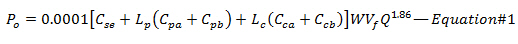We can solve the equation to get the flow rate (Q) that match with optimization criteria (Maximum Hydraulic Horsepower or Maximum Impact Force)

Maximum Hydraulic Horsepower will be happened when the pressure drop across the bit is equal to 65% of total pressure loss; therefore, 35% of total pressure loss (Ps) occurs through the drill stem (Po). The equation will be like this Po = 0.35 x Ps. By substituting Po with 0.35Ps into the Equation#1, you can solve for the optimum flow rate which will give you the maximum hydraulic horsepower.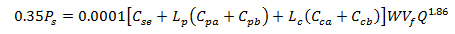Solve for Q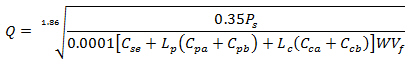Maximum Impact Force can be obtained when 48% of total pressure loss is at the bit so 52% of total pressure loss (Ps) occurs through the drill stem (Po). Substituting 52% of Ps into the Equation#1 will allow you to get the optimum flow rate at maximum impact force.Solve for Q## Coefficient For Annulus Around Drill Pipe and Drill Collar

A term “C” represents the general coefficient for annulus around drill pipe and drill collar and the equation is as follow: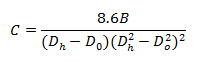Where:

Dh = hole diameter, inch

Do = Outside diameter of drill pipe or drill collar, inch

B = a parameter which takes into account the difference between Q1.86 and Q2

This term does not consider the different between size of tool joint and pipe which affect pressure drop in the annulus. This topic will demonstrate equations to get more accurate coefficient.

Coefficient of Annulus around Drill Pipe and Tool Joint

In order to get an accurate annulus pressure drop around a drill string, the coefficient for the annulus around tool joint must be taken in to account.